# Perimeter of Irregular Shapes

Perimeter of Irregular Shapes• A regular shape is a shape in which every side is the same length.
• If a shape is not regular then it is irregular.
• An irregular shape is simply a shape where not every single side is the same length.
• If a shape is irregular then it has angles that are not all the same size.
• To find the perimeter of an irregular shape, we simply add up each of its outer sides.
• Perimeter is a measurement of the total lengths and so the will be a length measurement (e.g. centimetres).
• We decide which irregular shape we have based on the number of sides or corners that it has.
• For example an irregular triangle has three sides and an irregular pentagon has five sides.
An irregular shape does not have sides that are all of equal length.

To find the perimeter of an irregular shape we add up all of the lengths of its outer sides.

#### Naming Irregular Shapes Summary Sheet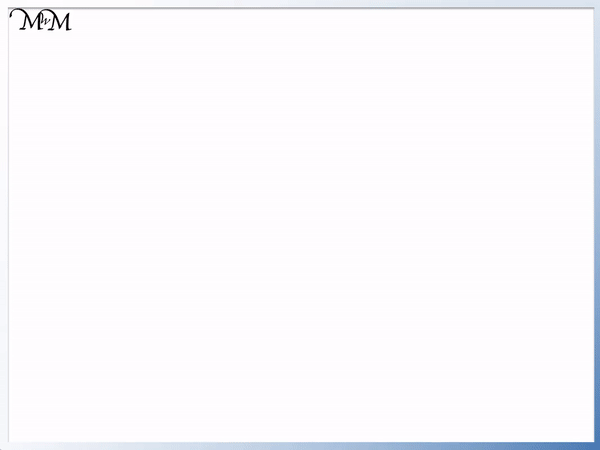• This shape is a because it has four sides.
• Even though two of its sides are both 5 cm, it is an irregular quadrilateral because not all of its sides are the same length.
• To find the perimeter of this irregular shape, we add up the four side lengths.
• We make the calculation easier by starting with the largest sides and also looking for .
• The two largest sides are 14 cm and 12 cm. These add together to make 26 cm.
• The two remaining sides are 5 cm and 5 + 5 = 10, using number bonds.
• 26 cm + 10 cm = 36 cm.
• The perimeter of this irregular quadrilateral is 36 cm.# How to Find the Perimeter of Irregular Shapes

To find the perimeter of an irregular shape you simply add up the outer side lengths of the shape.

In this lesson we will look at some examples of irregular shapes and learn how to calculate their perimeters.

A regular shape is a shape in which every side is the same length. All of its angles will also be the same size.

An irregular shape is simply any shape where not all of the side lengths and angles are the same. An irregular shape is any shape that is not regular.

We name irregular shapes based on how many sides they have. Since the number of corners a shape has is the same as the number of its sides, we can also name a shape by how many corners it has.

Below are some common examples of irregular shapes arranged in a list with their properties shown.When teaching irregular shapes it is important to be aware of a common mistake. People sometimes think that for a shape to be irregular, all of its sides have to be different to each other. However, a shape is irregular if it has at least one side that is of a different length to any of the other sides.

For example, a triangle can have two sides that are the same, but just one side that is different to the other two sides and it is an irregular shape.

Below are some examples of irregular shapes.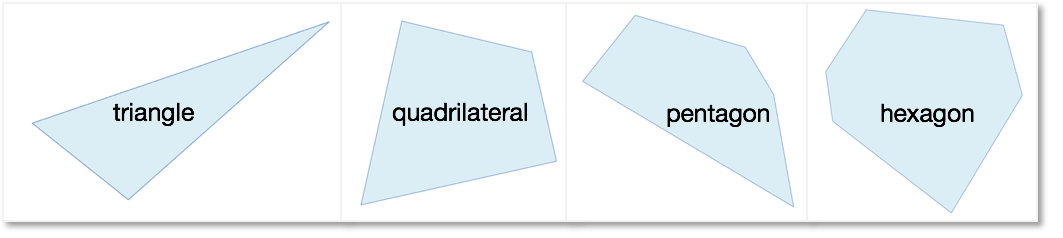The perimeter of a shape is the total distance around its outside edges.

To calculate the perimeter of a shape, we add the lengths of each side.

Here is an example of finding the perimeter of an irregular triangle: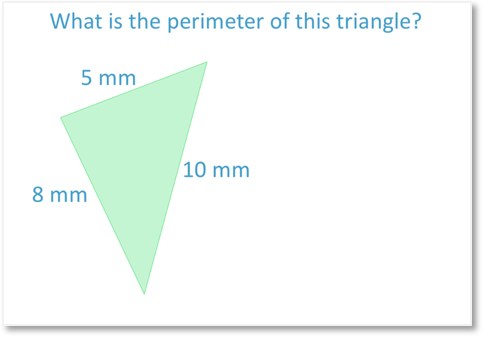To find the perimeter of this irregular triangle, we add up the three side lengths.

A tip for teaching perimeter of irregular shapes is to cross off each side length as you have added it.

A common mistake for children calculating perimeter is that they miss a side out completely or count a side more than once.

I recommend that to avoid this mistake, you could teach the child to pick a corner to start at. Move around the outside of the shape and cross off the sides as you add them if they are simple enough for your child to add. You could draw around the original shape as you go, or highlight each side, until you are back where you started.

However for some questions with larger numbers, this could result in calculations that are not always the easiest to do first. You could instead look for sides that add up to easier numbers, such as using number bonds and then just cross these sides off as you add them.

In this example, we only have three sides so we will just cross the sides off as we add them.8 + 10 = 18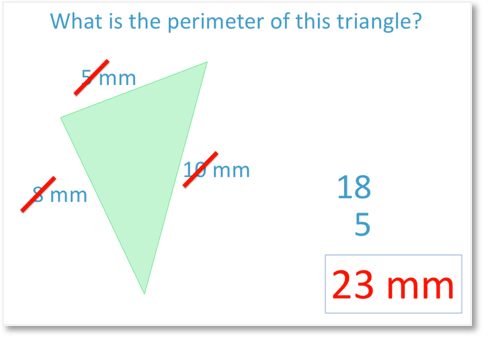18 + 5 = 23

The perimeter of this triangle is 23 mm.

Remember that perimeter is a measure of the total outside length of the shape and the answer that we get is a measurement of length.

Therefore our units of measurement are going to be length units. Since in our example we have measured each side in millimetres (mm), we have added up a total length in millimetres also.

We write an ‘mm’ after 23 to show that we have 23 millimetres. ‘mm’ is a short way of writing millimetres.

In the same way that we put a space between ’23’ and the word ‘millimetres’, we put a space in between ’23’ and ‘mm’. We wouldn’t write ’23millimetres’ we would write ’23 millimetres’ and in the same way we write ’23 mm’ not ’23mm’. This rule of leaving a space applies to all units of measurement.

Here’s another example of finding the perimeter of an irregular pentagon: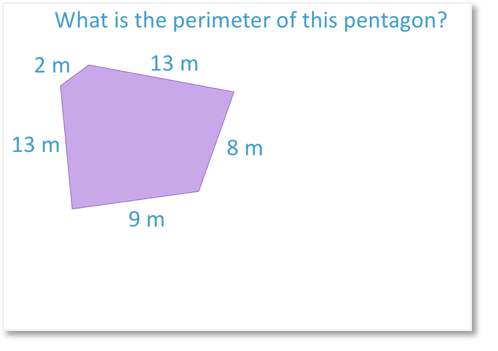As we said previously, this shape will be irregular because not all of its sides are the same.

Even though it has two sides that are the both 13 metres, it has three others sides that are all different to this. It is not regular, it is irregular.

To find the perimeter of this pentagon, we add the lengths of each side together.

To make sure that we don’t add a side more than once, we will cross out the sides when we add them.

We will also make sure that we write down our calculations to avoid making mistakes.

The side lengths are in metres. Instead of writing the word ‘metres’ next to every number, it is quicker and easier to write ‘m’. ‘m’ is the abbreviation of ‘metres’ and we write our number, then a space and then ‘m’.

We can start with the two largest sides, since these will not be particularly nice to add later to an already large number.

The two largest sides are both 13 metres long and because we have two of them, it means that we can double 13 to get our answer.

Doubling is generally an easier strategy to use than addition and it is another tip to look out for when approaching these perimeter calculations. We can look for any sides that are the same and multiply the length by how many sides have this length.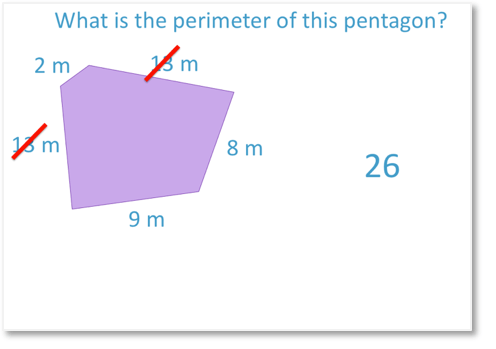13 + 13 = 26   (or 13 x 2 = 26)

Next we can see that we have a number bond to 10. We have 2 m and 8 m.

We can add these two sides together first.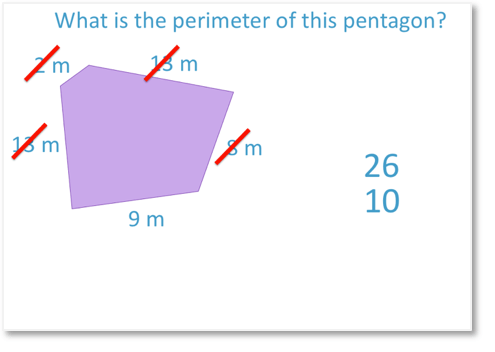8 + 2 = 10

Ten is an easier number to work with and to add. Adding ten simply involves adding one more to our tens column. We try and look for pairs of sides that will add to make ten if we can.

Now we add our final side, 9 m.26 + 10 + 9 = 45

An easy way to do this calculation is that 10 + 9 is 19 and that adding 19 is the same as adding 20 and then subtracting 1.

26 + 10 + 9 is the same as 26 + 19, which is the same as 26 + 20 then subtract 1.

26 + 20 = 46 and then we subtract one to compensate and we get 45 as we got before.

The perimeter of this irregular pentagon is 45 m.

The method for finding the perimeter is generally the same for all of our irregular shapes. We move around the outside of our shape and add up the outside edges, crossing them off as we add them.

Remember if there are multiple sides that are the same length, a strategy can be to use multiplication instead of adding them. We can also look for multiples of ten and number bonds to help with our addition, along with the strategy of adding with compensation if we have to add a number like 9 or 19.Now try our lesson on Perimeter of a Regular Shape where we learn a shortcut to find the perimeter of regular shapes.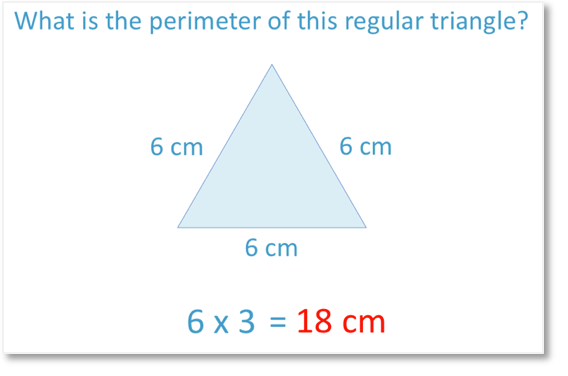error: Content is protected !!